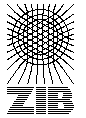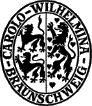# Testset P4Z

These instances are random generated complete graphs with random weights. The were introduced in CGR92 .

Name   |V|    |E|    |T|    DC    Opt
P401100 4950 Ps 155
P402100 4950 Ps 116
P403100 4950 Ps 179
P404100 4950 10 Ls 270
P405100 4950 10 Ls 270
P406100 4950 10 Ps 290
P407100 4950 20 Ps 590
P408100 4950 20 Ls 542
P409100 4950 50 Ps 963
P410100 4950 50 Ls 1010

The column DC classifies the difficulty of the instance.

L
Solvable by usage of local preprocessing. Typical examples are the SD-Test, BD-n Tests and FST computations. Neither a global upper nor lower bound needs to be computed.
P
Solvable by polynomial time algorithms, like dual ascent in combination with primal heuristic, a integral LP formulation or advanced preprocessing like reduced cost criteria or the RCR-Test.
NP
No polynomial time algorithm is known. Use of an exponential time enumeration sceme like Branch-and-Bound is neccessary.

The letter after class gives an impression how long it takes to solve the problem using state-of-the-art soft- and hardware. secounds means less than a minute (this includes instances which can be solved in fractions of a second). minutes means less than an hour. hours is less than a day and days is less than a week. weeks mean it takes really a long time to solve this instance. ? means the instance is not solved or the time is not known.

If the number in the Opt column is written in italics the optimum is not known. The number given is the best know upper bound.

Last Update : 2015/02/11 11:57:20 \$ by Thorsten Koch